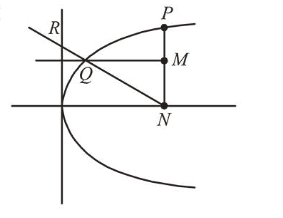# Let P be a point on the parabola,Question:

Let $P$ be a point on the parabola, $y^{2}=12 x$ and $N$ be the foot of the perpendicular drawn from $P$ on the axis of the parabola. A line is now drawn through the mid-point $M$ of $P N$, parallel to its axis which meets the parabola at $Q$. If the

$y$-intercept of the line $N Q$ is $\frac{4}{3}$, then :

1. (1) $P N=4$

2. (2) $M Q=\frac{1}{3}$

3. (3) $M Q=\frac{1}{4}$

4. (4) $P N=3$

Correct Option: , 3

Solution:$\because y^{2}=12 x$

$\therefore a=3$

Let $P\left(a t^{2}, 2 a t\right)$

$\Rightarrow N\left(a t^{2}, 0\right) \Rightarrow M\left(a t^{2}, a t\right)$

$\because$ Equation of $Q M$ is $y=a t$

So, $y^{2}=4 a x \Rightarrow x=\frac{a t^{2}}{4}$

$\Rightarrow Q\left(\frac{a t^{2}}{4}, a t\right)$

$\Rightarrow$ Equation of $Q N$ is $y=\frac{-4}{3 t}\left(x-a t^{2}\right)$

$\because Q N$ passes through $\left(0, \frac{4}{3}\right)$, then

$\frac{4}{3}=-\frac{4}{3 t}\left(-a t^{2}\right) \Rightarrow a t=1 \Rightarrow t=\frac{1}{3}$

Now, $M Q=\frac{3}{4} a t^{2}=\frac{1}{4}$ and $P N=2 a t=2$